## Pre-population format

Pre-population makes it possible to fill out responses to questions before the respondent views the survey form. It is possible to use pre-population by adding parameters to the survey URL or by adding attribute to invitee data. Both URLs and invitee data use the same format.

All pre-populated responses are based on parameters name and parameter value pairs. The parameter name identifies which question to pre-populate and the value specifies the response. The format of the value defines how to populate a multi-value response (for example a multiple choice or a matrix question).

Parameter name and value format:

• Rating:

Examples:

```        pre_q1=2

```

Description:

The rating parameter has a single value. N/A has the value 0.

The general format for rating question type is:

```        pre_q[question_number]=[value]

```
• Multiple choice:

Examples:

```        Multiple selection off: pre_q1=4
Multiple selection on: pre_q2=1-3-5
Multiple selection on: pre_q3=1-3-5(othertext)

```

Description:

The multiple choice question type can have multiple selection on or off. When multiple selection is off the parameter can have only one value, otherwise it can have multiple values separated by “-”. To pre-populate the “other” field, add text in parentheses. “Other” field can only be used with the last choice. If some of the text value contains the “-” character it must be preceded by “'” escape character (single quote).

The general format for multiple choice fields is:

```        pre_q[question_number]=[indexvalue-indexvalue-indexvalue-...-indexvalue(othertext)]

```
• Numeric:

Examples:

```        Integer: pre_q1=100
Decimal: pre_q2=5.75

```

Description:

Numeric value can be integer or decimal. There can be only one value for this parameter type.

The general format for numeric question type is:

```        pre_q[question_number]=[value]

```
• Dropdown:

Examples:

```        pre_q1=itemtext
pre_q2=itemtext1-itemtext4

```

Description:

The dropdown question type can have multiple selection on or off. When multiple selection is off the parameter can have only one value, otherwise it can have multiple values separated by “-”. The pre-populated text must match the dropdown item value, not the label.

The general format for dropdown question type parameter is:

```        pre_q[question_number]=[itemvalue-itemvalue-itemvalue-...]

```
• Matrix:

Examples: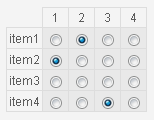```        Rating matrix group type: pre_q1=2-1--3

```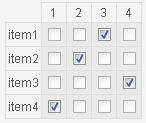```        Ranking matrix group type: pre_q1=3-2-4-1

```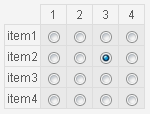```        Intersection matrix group type: pre_q1=7

```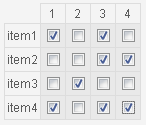```        Any cell matrix group type: pre_q1=1010001101001011

```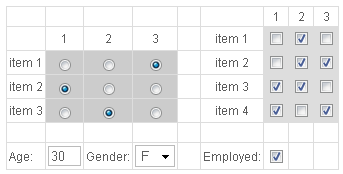```        Advanced matrix: pre_q1=010011110101-3-1-2-30-F-1

```

Description:

A matrix may contain many input values and types. Each value is separated by “-”. The number of parameters is the same as the number of input values. Cells with no input are empty cell, labels, images. For example, a text field, dropdown list, checkbox or a rating value are all input types. The matrix input fields are traversed from top-left to bottom-right, with the exception of cell groups. They are traversed independently like a matrix within the matrix. The number of values within a group depends on the type. Rating and ranking has one index value per row, intersection has a single index value, a group with checkboxes has a 0 or 1 value per cell. If no values are specified for some cells, separators must still exist.

The general format for matrix is:

```        pre_q[question_number]=[value-value-value-...]

```

(value represents a cell or group value)

• In-text fields:

Examples:

```        Text field: pre_q1_intxt=mytext
Numeric field integer: pre_q2_intxt=20
Numeric field decimal: pre_q3_intxt=17.45
Dropdown list: pre_q4_intxt=itemtext
Checkbox: pre_q5_intxt=1
Multiple values: pre_q6_intxt=mytext-20-17.45-itemtext-1

```

Description:

There can be unlimited number of in-text fields per question. Each value is separated by “-”. If some of the text value contains the “-” character it must be preceded by “'” escape character (single quote).

The general format for intext fields is:

```        pre_q[question_number]_intxt=[value-value-value-...]

```
• Free text:

Example:

```        pre_q1_ftxt=mytext

```

Description:

A single text value only can be specified per question for the free text input box.

The general format for free text is:

```        pre_q[question_number]_ftxt=[text]

```

All text values for all input types might contain the value separator “-”. To prevent Opinio interpreting this as a value separator, it can be escaped by a single quote preceding it.

The format described above is for pre-population using URL parameters. Some characters are not valid in a URL, because they are reserved as special URL characters. Examples are /,?,&,#. In order to get around this problem all URL parameters must be URL-encoded. See Wikipedia for more information.

Pre-population can also be done using invitations and custom data. Example of a CSV file for importing invitees:

```        email, pre_q1, pre_q2, pre_q2_ftxt
myemail@mail.com, 2, 1, mytext
youremail@mail.com, 4, 3, yourtext
heremail@mail.com, 8, 6, hertext

```

As you can see the parameter names are specified in the first line and the values are from the second line. The format of each value is the same as for URL parameters.

Using this format, it is possible to pre-populate the survey form with individual values per respondent. See the section called “Invitee data format” for more information on the invitee format.